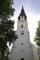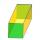# Billiard balls

A layer of ivory billiard balls of radius 6.35 cm is in the form of a square. The balls are arranged so that each ball is tangent to every one adjacent to it. In the spaces between sets of 4 adjacent balls other balls rest, equal in size to the original. These balls form in turn a second layer on top of the first. Successive layers of this sort form a pyramidal pile with a single ball resting on top. If the bottom layer contains 16 balls, what is the height of the pile.

Result

h =  39.641 cm

#### Solution:Leave us a comment of example and its solution (i.e. if it is still somewhat unclear...):

Showing 0 comments:Be the first to comment!#### To solve this example are needed these knowledge from mathematics:

Pythagorean theorem is the base for the right triangle calculator.

## Next similar examples:

1. Tangent spheresA sphere with a radius of 1 m is placed in the corner of the room. What is the largest sphere size that fits into the corner behind it? Additional info: Two spheres are placed in a corner of a room. The spheres are each tangent to the walls and floor and
2. Cube in sphereThe sphere is inscribed cube with edge 8 cm. Find the radius of the sphere.
3. Add vectorGiven that P = (5, 8) and Q = (6, 9), find the component form and magnitude of vector PQ.
4. Circle annulusThere are 2 concentric circles in the figure. Chord of larger circle 10 cm long is tangent to the smaller circle. What are does annulus have?
5. Third memberDetermine the third member of the AP if a4=93, d=7.5.
6. GP membersThe geometric sequence has 10 members. The last two members are 2 and -1. Which member is -1/16?
7. Parametric equationFind the parametric equation of a line with y-intercept (0,-4) and a slope of -2.
8. Tetrahedral pyramidIt is given a regular tetrahedral pyramid with base edge 6 cm and the height of the pyramid 10 cm. Calculate the length of its side edges.
9. Church roofThe roof of the church tower has the shape of a regular tetrahedral pyramid with base edge length 5.4 meters and a height 5 m. It was found that needs to be corrected 27% covering of the roof area. What amount of material will be required?
10. CardsSuppose that are three cards in the hats. One is red on both sides, one of which is black on both sides, and a third one side red and the second black. We are pulled out of a hat randomly one card and we see that one side of it is red. What is the probabi
11. Theorem proveWe want to prove the sentense: If the natural number n is divisible by six, then n is divisible by three. From what assumption we started?
12. Euclid2In right triangle ABC with right angle at C is given side a=27 and height v=12. Calculate the perimeter of the triangle.
13. Vector 7Given vector OA(12,16) and vector OB(4,1). Find vector AB and vector |A|.
14. ABS CNCalculate the absolute value of complex number -15-29i.
15. The ditchDitch with cross section of an isosceles trapezoid with bases 2m 6m are deep 1.5m. How long is the slope of the ditch?
16. 4s pyramidRegular tetrahedral pyramid has a base edge a=17 and collaterally edge length b=32. What is its height?
17. PrismThe lenght, width and height of a right prism are 6, 17 and 10 respectively. What is the lenght of the longest segment whose endpoints are vertices of the prism?